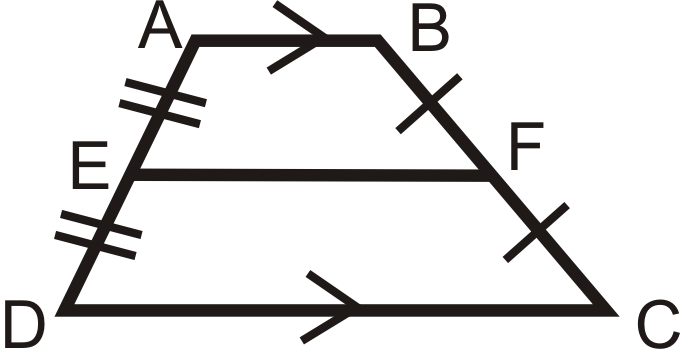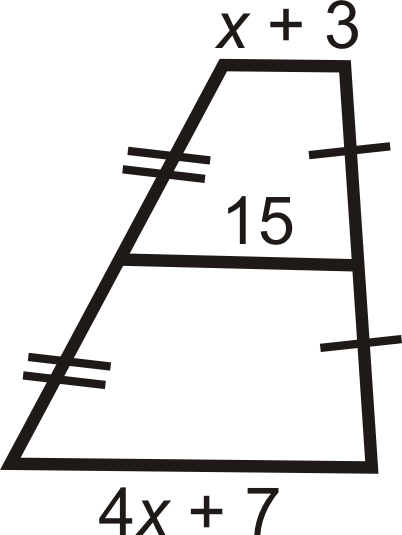# 5.13: Trapezoids

•• Contributed by CK12
• CK12

Determine unknown angle measurements of quadrilaterals with exactly one pair of parallel sides.

A trapezoid is a quadrilateral with exactly one pair of parallel sides.Figure $$\PageIndex{1}$$

An isosceles trapezoid is a trapezoid where the non-parallel sides are congruent.Figure $$\PageIndex{2}$$

The base angles of an isosceles trapezoid are congruent. If $$\(ABCD$$\) is an isosceles trapezoid, then $$\angle A\cong \angle B$$ and $$\angle C\cong \angle D$$.Figure $$\PageIndex{3}$$

The converse is also true. If a trapezoid has congruent base angles, then it is an isosceles trapezoid. The diagonals of an isosceles trapezoid are also congruent. The midsegment (of a trapezoid) is a line segment that connects the midpoints of the non-parallel sides:Figure $$\PageIndex{4}$$

There is only one midsegment in a trapezoid. It will be parallel to the bases because it is located halfway between them.

Midsegment Theorem: The length of the midsegment of a trapezoid is the average of the lengths of the bases.Figure $$\PageIndex{5}$$

If $$\overline{EF}$$ is the midsegment, then $$EF=\dfrac{AB+CD}{2}$$.

What if you were told that the polygon $$ABCD$$ is an isosceles trapezoid and that one of its base angles measures $$38^{\circ}$$? What can you conclude about its other base angle?

For Examples 1 and 2, use the following information:

$$\(TRAP$$\) is an isosceles trapezoid.Figure $$\PageIndex{6}$$

Example $$\PageIndex{1}$$

Find $$m\angle TPA$$.

Solution

$$\angle TPZ\cong \angle RAZ$$ so $$m\angle TPA=20^{\circ} +35^{\circ} =55^{\circ}$$.

Example $$\PageIndex{2}$$

Find $$m\angle ZRA$$.

Solution

Since $$m\angle PZA=110^{\circ}$$, $$m\angle RZA=70^{\circ}$$ because they form a linear pair. By the Triangle Sum Theorem, $$m\angle ZRA=90^{\circ}$$.

Example $$\PageIndex{3}$$

Look at trapezoid $$TRAP$$ below. What is $$m\angle A$$?Figure $$\PageIndex{7}$$

Solution

$$TRAP$$ is an isosceles trapezoid. $$m\angle R=115^{\circ}$$ also.

To find m\angle , set up an equation. \(\begin{aligned} 115^{\circ}+115^{\circ}+m \angle A+m \angle P &=360^{\circ} \\ 230^{\circ}+2 m \angle A &=360^{\circ} \quad \rightarrow m \angle A=m \angle P \\ 2 m \angle A &=130^{\circ} \\ m \angle A &=65^{\circ} \end{aligned}

Notice that $$m\angle R+m\angle A=115^{\circ} +65^{\circ} =180^{\circ}$$. These angles will always be supplementary because of the Consecutive Interior Angles Theorem.

Example $$\PageIndex{4}$$

Is $$ZOID$$ an isosceles trapezoid? How do you know?Figure $$\PageIndex{8}$$

Solution

$$40^{\circ} \neq 35^{\circ}$$, $$ZOID$$ is not an isosceles trapezoid.

Example $$\PageIndex{5}$$

Find $$x$$. All figures are trapezoids with the midsegment marked as indicated.

1.Figure $$\PageIndex{10}$$
1.Figure $$\PageIndex{10}$$
2.Figure $$\PageIndex{11}$$

Solution

1. $$x$$ is the average of 12 and 26. $$\dfrac{12+26}{2}=\dfrac{38}{2}=19$$
2. 24 is the average of $$x$$ and 35.

\begin{aligned} \dfrac{x+35}{2}&=24 \\ x+35&=48 \\ x&=13 \end{aligned}

1. 20 is the average of $$5x−15$$ and $$2x−8$$.

\begin{aligned} \dfrac{5x−15+2x−8}{2}&=20 \\ 7x−23&=40 \\ 7x&=63 \\ x&=9 \end{aligned}

## Review

1. Can the parallel sides of a trapezoid be congruent? Why or why not?

For questions 2-8, find the length of the midsegment or missing side.

1.Figure $$\PageIndex{12}$$
2.Figure $$\PageIndex{13}$$
3.Figure $$\PageIndex{14}$$
4.Figure $$\PageIndex{15}$$
5.Figure $$\PageIndex{16}$$
6.Figure $$\PageIndex{17}$$

Find the value of the missing variable(s).

1.Figure $$\PageIndex{18}$$

Find the lengths of the diagonals of the trapezoids below to determine if it is isosceles.

1. A(−3,2), B(1,3), C(3,−1), D(−4,−2)
2. A(−3,3), B(2,−2), C(−6,−6), D(−7,1)

## Vocabulary

Term Definition
isosceles trapezoid An isosceles trapezoid is a trapezoid where the non-parallel sides are congruent.
midsegment (of a trapezoid) A line segment that connects the midpoints of the non-parallel sides.
trapezoid A quadrilateral with exactly one pair of parallel sides.
Diagonal A diagonal is a line segment in a polygon that connects nonconsecutive vertices
midsegment A midsegment connects the midpoints of two sides of a triangle or the non-parallel sides of a trapezoid.

Interactive Element

Video: Trapezoids Examples - Basic

Activities: Trapezoids Discussion Questions

Study Aids: Trapezoids and Kites Study Guide

Practice: Trapezoids

Real World: Trapezoids in Timbuktu